Successfully reported this slideshow.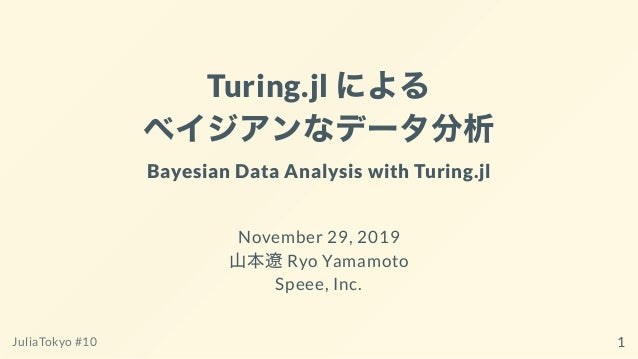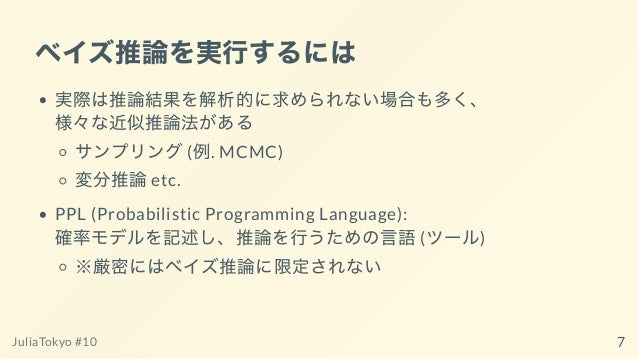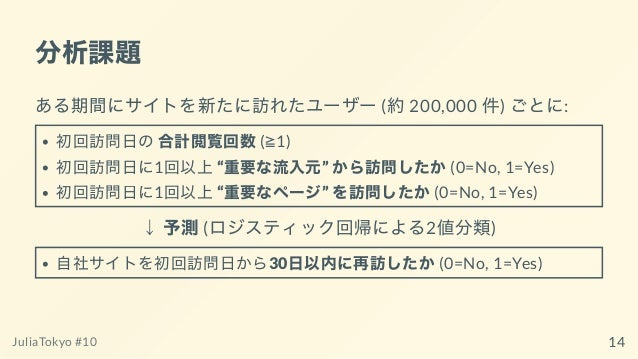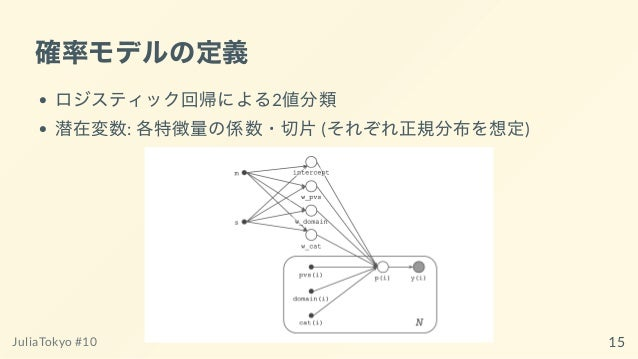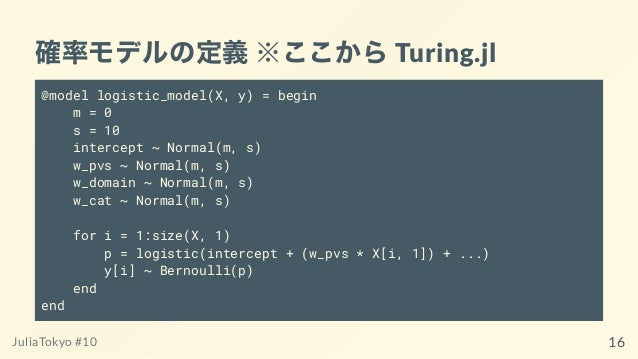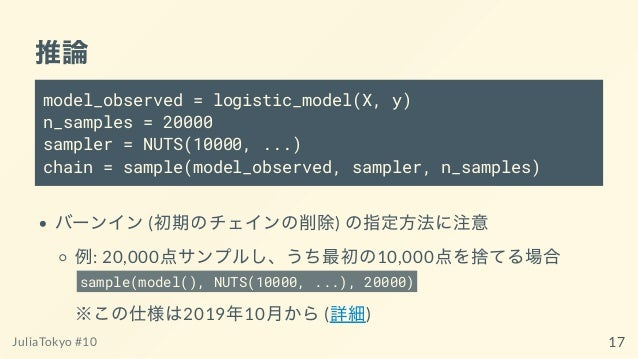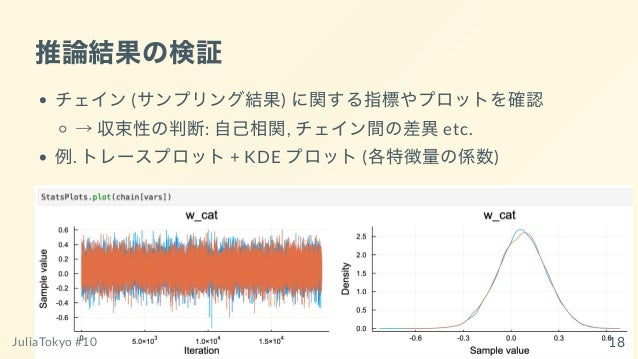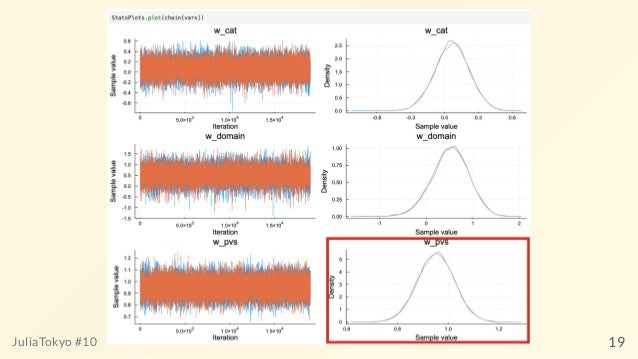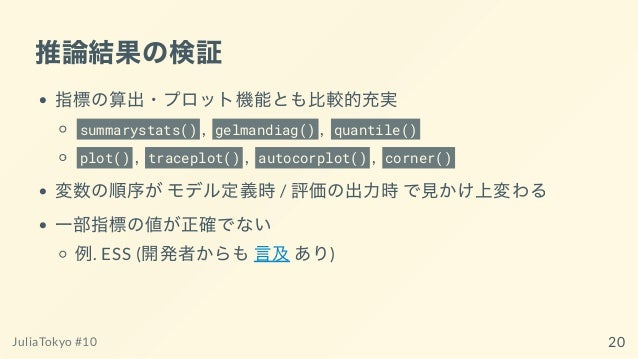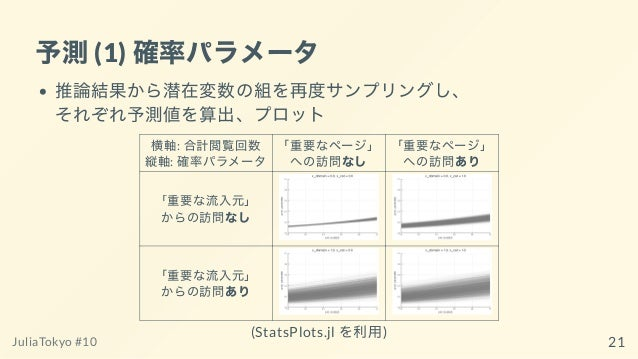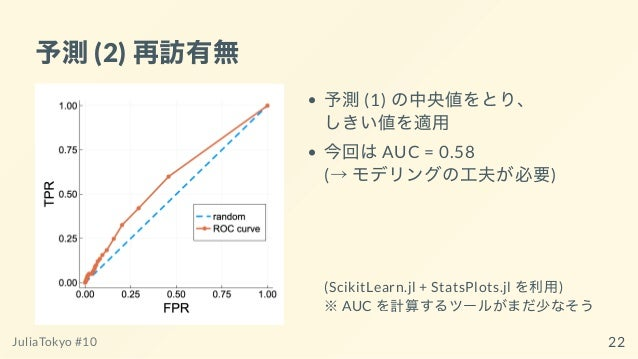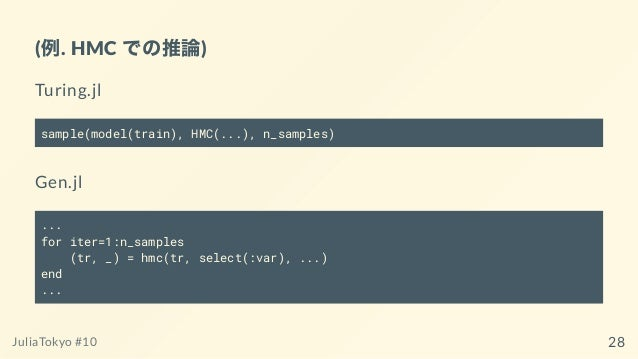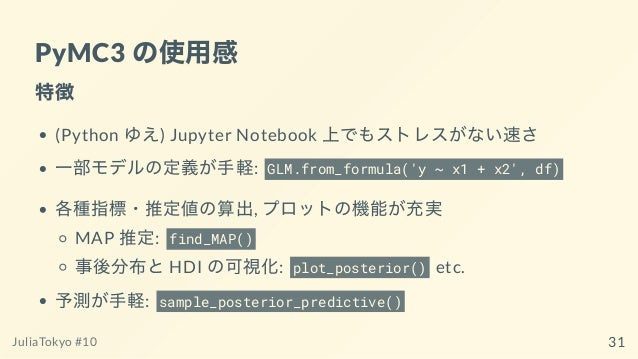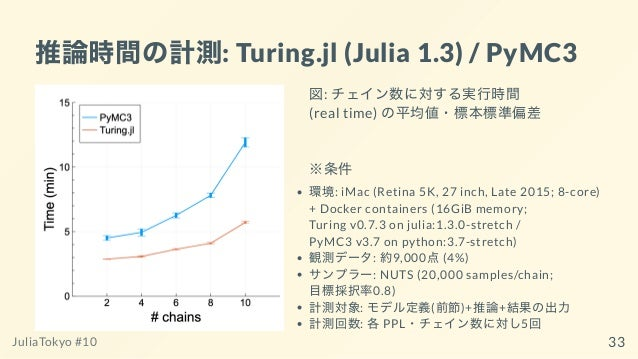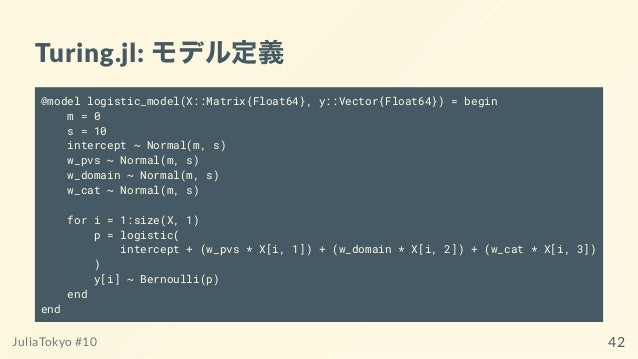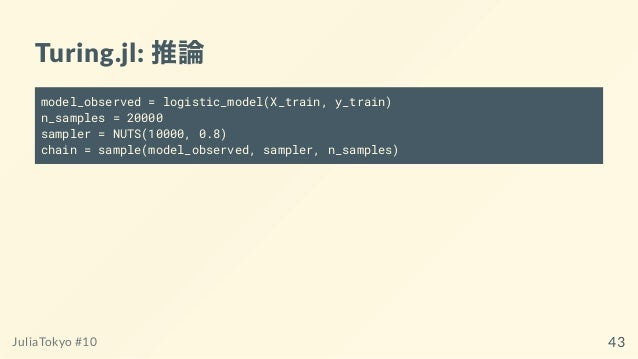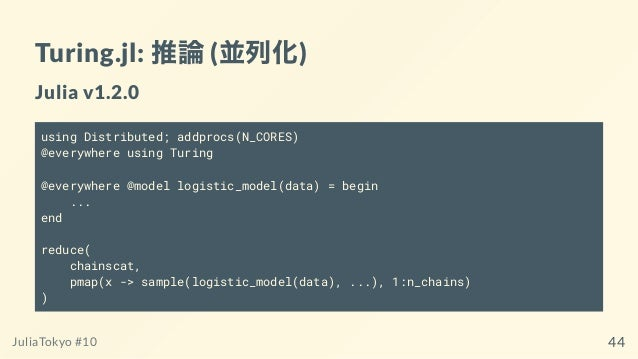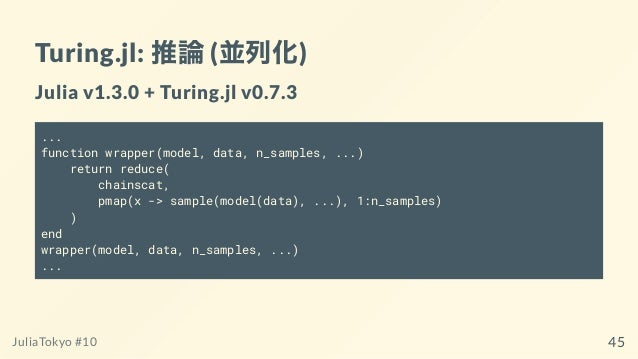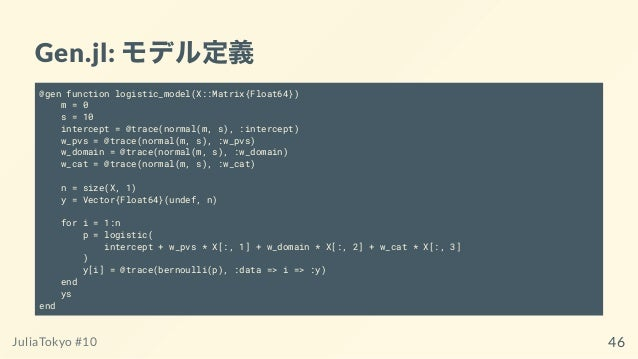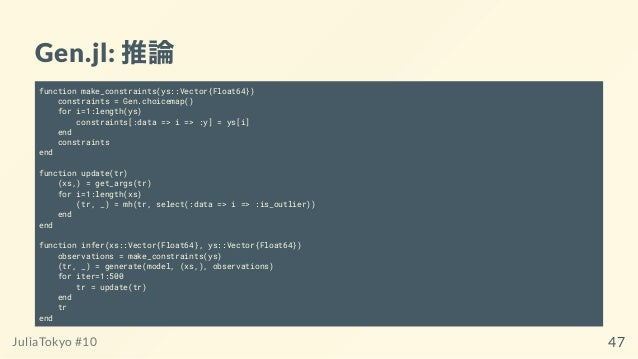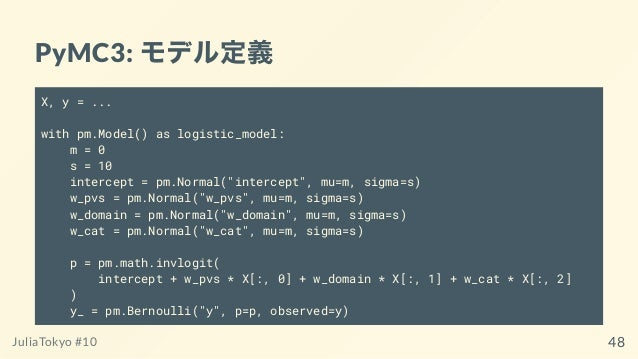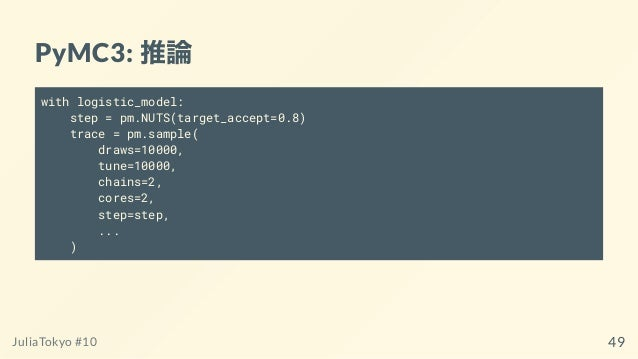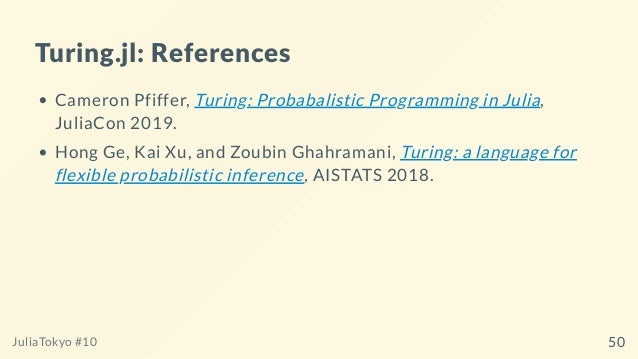Upcoming SlideShare
×
• Full Name
Comment goes here.

Are you sure you want to Yes No• Be the first to comment

### Turing.jl によるベイジアンなデータ分析 Bayesian Data Analysis with Turing.jl (JuliaTokyo #10)

1. 1. Turing.jl による ベイジアンなデータ分析 Bayesian Data Analysis with Turing.jl November 29, 2019 ⼭本遼Ryo Yamamoto Speee, Inc. JuliaTokyo #10 1
2. 2. ⾃⼰紹介  ⼭本遼Ryo Yamamoto  GitHub: @ryoyam  Twitter: @ryoyam11 データサイエンティスト/ ビジネストランスレーター@ Speee, Inc. 「PAAM」(データ活⽤によるマーケティング⽀援サービス) で 主にPython を利⽤してデータ分析を⾏っています Julia でのデータ分析ツール・事例が増えているトレンドを踏まえ、 状況に応じて導⼊を検討できるよう調査・使⽤中です JuliaTokyo #10 2
3. 3. 本⽇お話しすること はじめに: ベイズ推論とPPL の概要 Turing.jl によるビジネスデータの分析例 その他のPPL との使⽤感の⽐較 おわりに: 実⽤的観点からの所感 JuliaTokyo #10 3
4. 4. 本⽇お話しすること はじめに: ベイズ推論とPPL の概要 Turing.jl によるビジネスデータの分析例 その他のPPL との使⽤感の⽐較 おわりに: 実⽤的観点からの所感 JuliaTokyo #10 4
5. 5. はじめに: ベイズ推論とPPL の概要 JuliaTokyo #10 5
6. 6. ベイズ推論の考え⽅ 確率を「事象に対する確信度」として扱い、 観測データに基づき更新していく(事前分布→事後分布) “データ⽣成の背後にある構造” (確率モデル) を表現し、 実際の観測データによって具体形を推し量ることができる 従来(non-Bayesian) の機械学習と⽐べ、得られる情報が多い: 従来: 最適化後の「点」 vs ベイズ推論: 潜在変数/観測変数の「分布」 JuliaTokyo #10 6
7. 7. ベイズ推論を実⾏するには 実際は推論結果を解析的に求められない場合も多く、 様々な近似推論法がある サンプリング(例. MCMC) 変分推論etc. PPL (Probabilistic Programming Language): 確率モデルを記述し、推論を⾏うための⾔語(ツール) ※厳密にはベイズ推論に限定されない JuliaTokyo #10 7
8. 8. PPL の選択肢 Stan, JAGS Python PyMC3 その他: Pyro, Edward2 etc. Julia Turing.jl Gen.jl その他: Mamba.jl etc. JuliaTokyo #10 8
9. 9. 本⽇お話しすること はじめに: ベイズ推論とPPL の概要 Turing.jl によるビジネスデータの分析例 その他のPPL との使⽤感の⽐較 おわりに: 実⽤的観点からの所感 JuliaTokyo #10 9
10. 10. Turing.jl による ビジネスデータの分析例 JuliaTokyo #10 10
11. 11. 例題 次のようなケースを考えます: あるEC サイトの運営者からの分析依頼 ⾃社ブランドを気に⼊ってくれそうな潜在顧客に 早期からアプローチし、購⼊につなげたい そこで、⾃社サイトの「アクセスログ」を使って、 どのようなユーザーであれば再訪してくれるか (傾向, 条件) を知りたい JuliaTokyo #10 11
12. 12. 利⽤可能なデータ(アクセスログ) ユーザーがWeb サイトを訪れた際、 ブラウザごとに⼀意なID を発⾏して 「訪問時刻」 「訪問したページのURL」 「流⼊元(訪問直前に閲覧していた外部Web サイト) のURL 」 などを記録したもの 2回⽬以降の訪問時は、初回訪問時のID で同様に記録し、 ユーザーの閲覧⾏動を追跡できる JuliaTokyo #10 12
13. 13. 分析にあたって置く仮説 訪問初⽇の合計閲覧回数が多いユーザーほど、 再訪しやすいのでは？ ⾃社ブランドと関連性が⾼い流⼊元(“重要な流⼊元”) から やってくるユーザーほど、再訪しやすいのでは？ 例. Twitter の公式アカウントのページ ⾃社ブランドについて詳しく紹介したページ(“重要なページ”) を 訪れるユーザーほど、再訪しやすいのでは？ 例: 個別商品に関するページ JuliaTokyo #10 13
14. 14. 分析課題 ある期間にサイトを新たに訪れたユーザー(約200,000 件) ごとに: 初回訪問⽇の合計閲覧回数(≧1) 初回訪問⽇に1回以上“重要な流⼊元” から訪問したか(0=No, 1=Yes) 初回訪問⽇に1回以上“重要なページ” を訪問したか(0=No, 1=Yes) ↓予測(ロジスティック回帰による2値分類) ⾃社サイトを初回訪問⽇から30⽇以内に再訪したか(0=No, 1=Yes) JuliaTokyo #10 14
15. 15. 確率モデルの定義 ロジスティック回帰による2値分類 潜在変数: 各特徴量の係数・切⽚(それぞれ正規分布を想定) JuliaTokyo #10 15
16. 16. 確率モデルの定義※ここからTuring.jl @model logistic_model(X, y) = begin m = 0 s = 10 intercept ~ Normal(m, s) w_pvs ~ Normal(m, s) w_domain ~ Normal(m, s) w_cat ~ Normal(m, s) for i = 1:size(X, 1) p = logistic(intercept + (w_pvs * X[i, 1]) + ...) y[i] ~ Bernoulli(p) end end JuliaTokyo #10 16
17. 17. 推論 model_observed = logistic_model(X, y) n_samples = 20000 sampler = NUTS(10000, ...) chain = sample(model_observed, sampler, n_samples) バーンイン(初期のチェインの削除) の指定⽅法に注意 例: 20,000点サンプルし、うち最初の10,000点を捨てる場合 sample(model(), NUTS(10000, ...), 20000) ※この仕様は2019年10⽉から(詳細) JuliaTokyo #10 17
18. 18. 推論結果の検証 チェイン(サンプリング結果) に関する指標やプロットを確認 →収束性の判断: ⾃⼰相関, チェイン間の差異etc. 例. トレースプロット+ KDE プロット(各特徴量の係数) JuliaTokyo #10 18
19. 19. JuliaTokyo #10 19
20. 20. 推論結果の検証 指標の算出・プロット機能とも⽐較的充実 summarystats() , gelmandiag() , quantile() plot() , traceplot() , autocorplot() , corner() 変数の順序がモデル定義時/ 評価の出⼒時で⾒かけ上変わる ⼀部指標の値が正確でない 例. ESS (開発者からも⾔及あり) JuliaTokyo #10 20
21. 21. 予測(1) 確率パラメータ 推論結果から潜在変数の組を再度サンプリングし、 それぞれ予測値を算出、プロット 横軸: 合計閲覧回数 縦軸: 確率パラメータ 「重要なページ」 への訪問なし 「重要なページ」 への訪問あり 「重要な流⼊元」 からの訪問なし 「重要な流⼊元」 からの訪問あり (StatsPlots.jl を利⽤) JuliaTokyo #10 21
22. 22. 予測(2) 再訪有無 予測(1) の中央値をとり、 しきい値を適⽤ 今回はAUC = 0.58 (→モデリングの⼯夫が必要) (ScikitLearn.jl + StatsPlots.jl を利⽤) ※AUC を計算するツールがまだ少なそう JuliaTokyo #10 22
23. 23. 予測 予測をワンストップで⾏う機能はない 「潜在変数の事後分布のサンプリング」+「予測値の算出」 を順に⾏う必要がある JuliaTokyo #10 23
24. 24. 本⽇お話しすること はじめに: ベイズ推論とPPL の概要 Turing.jl によるビジネスデータの分析例 その他のPPL との使⽤感の⽐較 おわりに: 実⽤的観点からの所感 JuliaTokyo #10 24
25. 25. その他のPPL との使⽤感の⽐較 JuliaTokyo #10 25
26. 26. ⽐較対象 Turing.jl (分析例で紹介) v0.7.2, v0.7.3 Gen.jl v0.3.1 PyMC3 v3.7 JuliaTokyo #10 26
27. 27. Gen.jl の使⽤感 特徴 General-purpose = MCMC 以外の⼿法もカバー 確率モデルの定義: 他ツールと似た構⽂ 推論: low-level な部分をカスタマイズできる サンプル1回ごとに値の更新⽅法(update) の指定 提案分布(proposal) の指定etc. JuliaTokyo #10 27
28. 28. (例. HMC での推論) Turing.jl sample(model(train), HMC(...), n_samples) Gen.jl ... for iter=1:n_samples (tr, _) = hmc(tr, select(:var), ...) end ... JuliaTokyo #10 28
29. 29. Gen.jl の使⽤感 注意点 「更新」「観測データの設定」のコードを毎度書く必要がある 更新⽅法を⾃動的にチューニングする機能はない 例. HMC はあるがNUTS はない まだpre-release →現状では、実務で⼿軽に使いたい場合には向かない JuliaTokyo #10 29
30. 30. Gen.jl の使⽤感 注意点 実⽤⾯からは「あると嬉しい」機能が少ない: 収束性の検証のための機能 可視化の機能 GenViz.jl: 各更新におけるスナップショットを可視化できる が、traceplot, autocorplot などは出せない 予測(PPC) の機能 JuliaTokyo #10 30
31. 31. PyMC3 の使⽤感 特徴 (Python ゆえ) Jupyter Notebook 上でもストレスがない速さ ⼀部モデルの定義が⼿軽: GLM.from_formula('y ~ x1 + x2', df) 各種指標・推定値の算出, プロットの機能が充実 MAP 推定: find_MAP() 事後分布とHDI の可視化: plot_posterior() etc. 予測が⼿軽: sample_posterior_predictive() JuliaTokyo #10 31
32. 32. PyMC3 の使⽤感 注意点 PyMC3 はv3.7 で開発終了、PyMC4 がリリース予定(参考) ⼤幅な仕様変更の可能性も JuliaTokyo #10 32
33. 33. 推論時間の計測: Turing.jl (Julia 1.3) / PyMC3 図: チェイン数に対する実⾏時間 (real time) の平均値・標本標準偏差 ※条件 環境: iMac (Retina 5K, 27 inch, Late 2015; 8-core) + Docker containers (16GiB memory; Turing v0.7.3 on julia:1.3.0-stretch / PyMC3 v3.7 on python:3.7-stretch) 観測データ: 約9,000点(4%) サンプラー: NUTS (20,000 samples/chain; ⽬標採択率0.8) 計測対象: モデル定義(前節)+推論+結果の出⼒ 計測回数: 各PPL・チェイン数に対し5回 JuliaTokyo #10 33
34. 34. 補⾜ Turing.jl の場合の注意点 Julia v1.3.0 で並列実⾏しようとするとエラーとなった Github 上の回避策を使うと無事動作した JuliaTokyo #10 34
35. 35. 本⽇お話しすること はじめに: ベイズ推論とPPL の概要 Turing.jl によるビジネスデータの分析例 その他のPPL との使⽤感の⽐較 おわりに: 実⽤的観点からの所感 JuliaTokyo #10 35
36. 36. おわりに: 実⽤的観点からの所感 PPL の選択について 機能⾯: PyMC3 がカバレッジ・⼿軽さとも勝る 速度⾯: Turing.jl はPyMC3 と同等かそれ以上の⽔準 今後について Julia 製ツールの多くは今まさに発展途中という印象 実務で本格的に利⽤できる⽇が楽しみ JuliaTokyo #10 36
37. 37. 参考⽂献 Julia を学ぶにあたり、活⽤させていただきました: Kamiński et al., Julia プログラミングクックブック, O'Reilly, 2019 佐藤他, はじめてのJulia, WEB+DB PRESS vol. 111, 技術評論社, 2019 JuliaTokyo #10 37
38. 38. 本⽇お話しすること はじめに: ベイズ推論とPPL の概要 Turing.jl によるビジネスデータの分析例 その他のPPL との使⽤感の⽐較 おわりに: 実⽤的観点からの所感 JuliaTokyo #10 38
39. 39. 終 ありがとうございました JuliaTokyo #10 39
40. 40. JuliaTokyo #10 40
41. 41. Appendices JuliaTokyo #10 41
42. 42. Turing.jl: モデル定義 @model logistic_model(X::Matrix{Float64}, y::Vector{Float64}) = begin m = 0 s = 10 intercept ~ Normal(m, s) w_pvs ~ Normal(m, s) w_domain ~ Normal(m, s) w_cat ~ Normal(m, s) for i = 1:size(X, 1) p = logistic( intercept + (w_pvs * X[i, 1]) + (w_domain * X[i, 2]) + (w_cat * X[i, 3]) ) y[i] ~ Bernoulli(p) end end JuliaTokyo #10 42
43. 43. Turing.jl: 推論 model_observed = logistic_model(X_train, y_train) n_samples = 20000 sampler = NUTS(10000, 0.8) chain = sample(model_observed, sampler, n_samples) JuliaTokyo #10 43
44. 44. Turing.jl: 推論(並列化) Julia v1.2.0 using Distributed; addprocs(N_CORES) @everywhere using Turing @everywhere @model logistic_model(data) = begin ... end reduce( chainscat, pmap(x -> sample(logistic_model(data), ...), 1:n_chains) ) JuliaTokyo #10 44
45. 45. Turing.jl: 推論(並列化) Julia v1.3.0 + Turing.jl v0.7.3 ... function wrapper(model, data, n_samples, ...) return reduce( chainscat, pmap(x -> sample(model(data), ...), 1:n_samples) ) end wrapper(model, data, n_samples, ...) ... JuliaTokyo #10 45
46. 46. Gen.jl: モデル定義 @gen function logistic_model(X::Matrix{Float64}) m = 0 s = 10 intercept = @trace(normal(m, s), :intercept) w_pvs = @trace(normal(m, s), :w_pvs) w_domain = @trace(normal(m, s), :w_domain) w_cat = @trace(normal(m, s), :w_cat) n = size(X, 1) y = Vector{Float64}(undef, n) for i = 1:n p = logistic( intercept + w_pvs * X[:, 1] + w_domain * X[:, 2] + w_cat * X[:, 3] ) y[i] = @trace(bernoulli(p), :data => i => :y) end ys end JuliaTokyo #10 46
47. 47. Gen.jl: 推論 function make_constraints(ys::Vector{Float64}) constraints = Gen.choicemap() for i=1:length(ys) constraints[:data => i => :y] = ys[i] end constraints end function update(tr) (xs,) = get_args(tr) for i=1:length(xs) (tr, _) = mh(tr, select(:data => i => :is_outlier)) end end function infer(xs::Vector{Float64}, ys::Vector{Float64}) observations = make_constraints(ys) (tr, _) = generate(model, (xs,), observations) for iter=1:500 tr = update(tr) end tr end JuliaTokyo #10 47
48. 48. PyMC3: モデル定義 X, y = ... with pm.Model() as logistic_model: m = 0 s = 10 intercept = pm.Normal("intercept", mu=m, sigma=s) w_pvs = pm.Normal("w_pvs", mu=m, sigma=s) w_domain = pm.Normal("w_domain", mu=m, sigma=s) w_cat = pm.Normal("w_cat", mu=m, sigma=s) p = pm.math.invlogit( intercept + w_pvs * X[:, 0] + w_domain * X[:, 1] + w_cat * X[:, 2] ) y_ = pm.Bernoulli("y", p=p, observed=y) JuliaTokyo #10 48
49. 49. PyMC3: 推論 with logistic_model: step = pm.NUTS(target_accept=0.8) trace = pm.sample( draws=10000, tune=10000, chains=2, cores=2, step=step, ... ) JuliaTokyo #10 49
50. 50. Turing.jl: References Cameron Pfiffer, Turing: Probabalistic Programming in Julia, JuliaCon 2019. Hong Ge, Kai Xu, and Zoubin Ghahramani, Turing: a language for flexible probabilistic inference, AISTATS 2018. JuliaTokyo #10 50
51. 51. JuliaTokyo #10 51